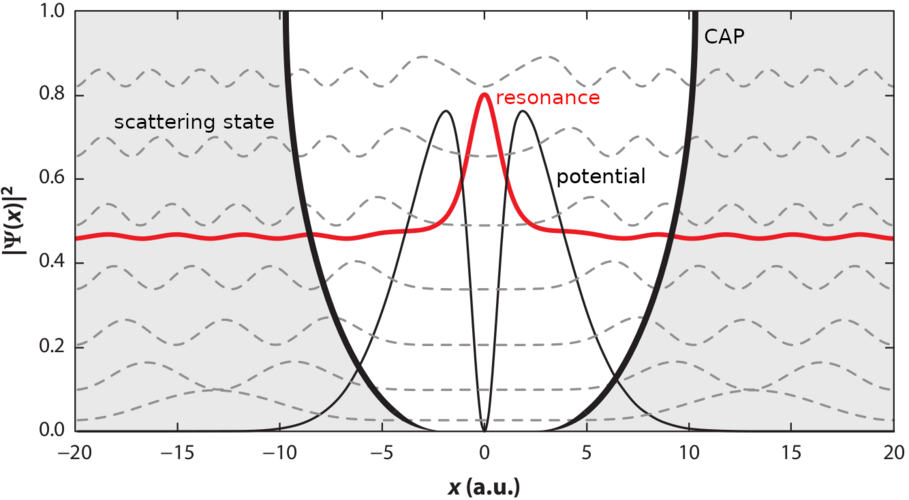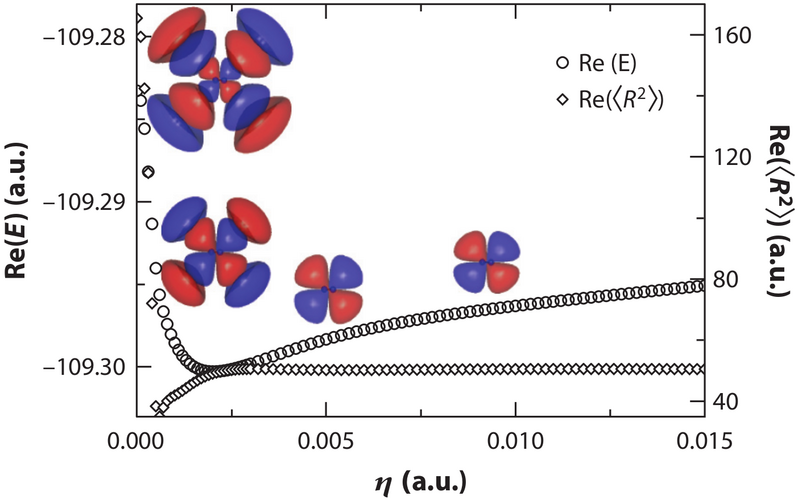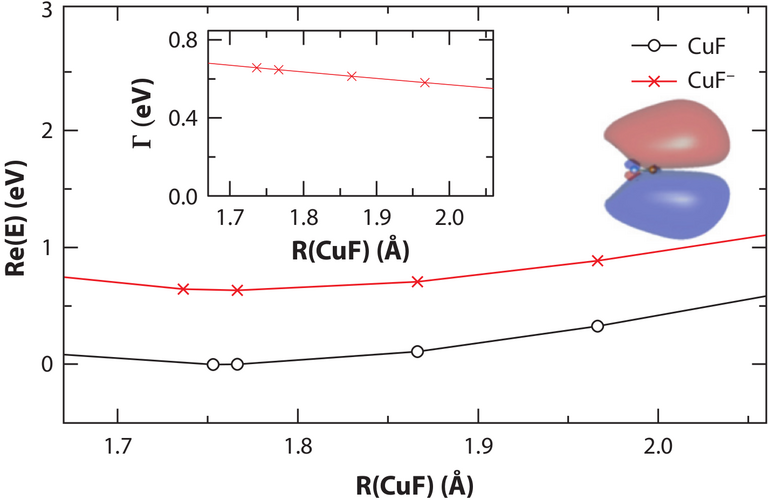# Calculating Electronic Resonances with Complex-Valued EOM-CC Methods• Resonances are electronically unbound states; they are metastable and decay by ejecting an electron.

• Resonances are embedded in the continuum and are not accessible by standard quantum-chemistry methods.

• Within non-Hermitian quantum mechanics, resonances become $$L^2$$-integrable, discrete states with complex energies (the real part describes resonance position and the imaginary part describes the resonance width); this enables calculation of resonances by standard quantum chemistry methods.

• Non-Hermitian extensions of quantum chemistry include 1) scaling all coordinates of the Hamiltonian with a complex factor exp(i*$$\theta$$) (the complex-scaling method); 2) using complex basis functions; 3) augmenting the Hamiltonian by a local complex absorbing potential i*$$\eta$$*W(r).

• EOM-CC methods provide an excellent platform for treating resonances because they efficiently include correlation and tackle multiple electronic states of different character in a balanced fashion.

• Energies, properties, and nuclear gradients are available for several complex-valued EOM-CC methods.Stabilization of the the 2$$\Pi_g$$ resonance of N$$_{2}^{-}$$ with increasing CAP intensity from EOM-EA-CCSD calculationsThe potential energy curve for the $$^2\Pi$$ resonance of CuF$$^{-}$$ from CAP-EOM-CCSD calculations